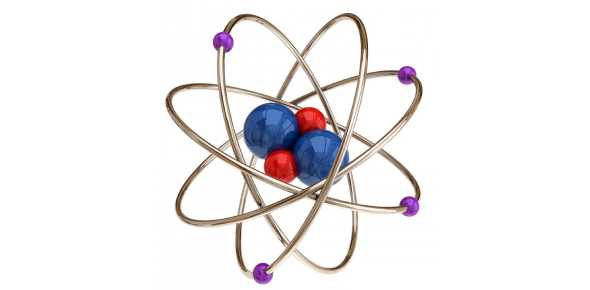# A Quiz On Atomic Theory: Trivia Quiz!

Approved & Edited by ProProfs Editorial Team
At ProProfs Quizzes, our dedicated in-house team of experts takes pride in their work. With a sharp eye for detail, they meticulously review each quiz. This ensures that every quiz, taken by over 100 million users, meets our standards of accuracy, clarity, and engagement.
| Written by Uvschubert
U
Uvschubert
Community Contributor
Quizzes Created: 1 | Total Attempts: 1,650
Questions: 9 | Attempts: 1,683SettingsAre you aware of atomic theory? Atomic theory is the scientific concept that matter is made up of atoms. According to this perspective, if you were to take a piece of matter and cut it into smaller fragments that are all the same size, it would come to a point where the pieces could not be cut into smaller pieces anymore. This quiz is fun because science is fun. Enjoy this wonderful quiz on atomic theory.

• 1.

### What scientist was the first to conclude through experimentation that atoms have positive charges in their nuclei?

• A.

Bohr

• B.

Balton

• C.

Mosley

• D.

Rutherford

D. Rutherford
Explanation
Rutherford discovered that atoms have a dense, positively charged nucleus through the gold foil experiment.

Rate this question:

• 2.

### Atoms of the same element must

• A.

Contain the same number of neutrons

• B.

Have the same mass number

• C.

Contain the same number of protons

• D.

Have equal numbers of protons and neutrons

C. Contain the same number of protons
Explanation
Atoms of the same element have the same atomic number (given on the periodic table), which is equal to the number of protons.

Rate this question:

• 3.

### Phosphorus: protons   neutrons   electrons

• A.

11 12 10

• B.

1 0 2

• C.

15 16 15

• D.

20 20 18

C. 15 16 15
Explanation
The atomic number is 15, which means phosphorus has 15 protons. The mass number is 31, which is the sum of protons plus neutrons. 31 - 15 = 16 neutrons. Because a neutral atom has equal numbers of protons and electrons, phosphorus also has 15 electrons.

Rate this question:

• 4.

### An increase in atomic number is related to an increase in atomic mass because -

• A.

More electrons are present in the atomic nucleus

• B.

More electrons are orbiting the atomic nucleus

• C.

More protons are present in the atomic nucleus

• D.

More protons are orbiting the atomic nucleus

C. More protons are present in the atomic nucleus
Explanation
If the atomic number has increased, that means protons have been added. Protons are located in the nucleus and have mass.

Rate this question:

• 5.

### How many protons, neutrons, and electrons are in a neutral atom of sodium?

• A.

11 p+, 12 n0, 11 e-

• B.

11 p+, 11 n0, 12 e-

• C.

12 p+, 11 n0, 12 e-

• D.

12 p+, 11 n0, 11 e-

A. 11 p+, 12 n0, 11 e-
Explanation
Sodium has an atomic number of 11, which means it has 11 protons. If the atom is neutral, it has equal numbers of protons and electrons. The mass number of sodium is 23; mass number - atomic number = neutrons. 23 - 11 = 12 neutrons

Rate this question:

• 6.

### Isotopes of an element have different -

• A.

Atomic numbers

• B.

Mass numbers

• C.

Numbers of protons

• D.

Numbers of outer-shell electrons

B. Mass numbers
Explanation
Isotopes of an element have different numbers of neutrons. The mass number is equal to the sum of the number of protons plus neutrons in the atom. If neutrons differ between atoms, mass number also differs.

Rate this question:

• 7.

### Radioactive iodine-131 has a half-life of eight days. The amount of a 200.0 gram sample left after 32 days would be

• A.

6.25 g

• B.

12.5 g

• C.

25.0 g

• D.

50.0 g

B. 12.5 g
Explanation
Each half life is eight days. In thirty two days, there are four half lives. As each half-life occurs, the amount of iodine-131 decreases by half. After dividing 200.0 g by 2 four times, the amount left is 12.5 g.

Rate this question:

• 8.

### Which of these conclusions can be drawn from Rutherford's experiment?

• A.

Each atom contains electrons.

• B.

The nucleus of an atom can be split.

• C.

Each atom contains protons.

• D.

Atoms are mostly empty space.

D. Atoms are mostly empty space.
Explanation
Rutherford's experiment involved firing alpha particles at a thin sheet of gold foil. He observed that most of the alpha particles passed through the foil without any deflection, while a small fraction were deflected at large angles. This led him to conclude that atoms are mostly empty space, with a tiny, dense nucleus at the center where most of the mass is concentrated. The fact that most of the alpha particles passed through the foil without being deflected indicated that atoms do not contain electrons, protons, or a nucleus that can be split.

Rate this question:

• 9.

### How does the radioactive isotope of C-14 differ from its stable counterpart C-12?

• A.

It has a different number of protons and two less neutrons than C-12.

• B.

It has the same number of protons and two more electrons than C-12.

• C.

It has the same number of protons but two more neutrons than C-12.

• D.

It has a different number of protons and two more neutrons than C-12.Back to top